Basic Electronics

# Inverting Amplifier and Circuit Operation

## Inverting Amplifier

The close-loop configuration of an operational amplifier, in which input signal is applied on inverting input, is called inverting amplifier (remember the term close-loop means re-connecting output with input through a feedback circuit) In other words, inverting amplifier is a close loop circuit of an operational amplifier, from which negative gain is received and which is detected through an external resistor. As amplifier name suggests, when a signal is applied on its inverting input, an inverted polarity amplified signal is received through its output (i.e. a difference of 180˚ or phase difference exists between the output received from such an amplifier and its input. As output voltage and input voltage are mutually 180˚ out of phase, that’s why such an amplifier is known as an inverted amplifier. Inverting amplifier is the most basic circuit of an operational amplifier, in which cumulative voltage gain is strengthened via application of negative feedback. As open loop voltage gain (AOL) is too high (i.e. even higher than 200,000) therefore, its application without any feedback is quite unsteady. Therefore, cumulative voltage gain requires to be strengthened via the application of negative feedback. Therefore, an inverting amplifier is also known as a negative scalar.

Figure 8.46 – inverting amplifierIn figure 8.46 an inverting amplifier has been illustrated. According to the figure, some part of the amplifiers’ output has been provided a feedback via inverting input’s resistance R2. However, input signal has also been applied on inverting input via resistance R1, whereas non-inverting input is normally grounded. Remember that output signal is received between output terminal and ground of an operational amplifier.

### Circuit Operation

For understanding the mechanism of an inverting amplifier, study the circuit shown in figure 8.47. Suppose that initial value of Vin is zero. As no potential difference exists between both input terminals during such a situation, therefore, output is also zero.

Figure 8.47- operation of an inverting amplifier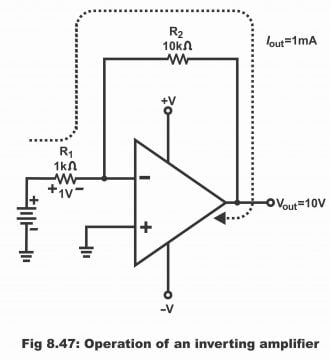Now if Vin is gradually increased to +1V, in such a situation input starts getting 1V. However, output of an operational amplifier tries to keep inverting input on zero (remember that potential of non- inverting input is also zero). If the attempt succeeds, voltage drop parallel to R1 will be 1 volt, the polarity of which has been illustrated in the figure. 1mA current passes through it due to the presence of 1 volt parallel to 1KΩ. As no current penetrates into inverting input due to high input impedance, therefore this 1mA current should logically be received via output. As R1 and R2 are mutually arranged in a series or they are mutually configured, therefore current which passes through R1, should also pass through R2. Thus, when 1ma current transmits through R2, a drop of 10V will be received parallel to it. As potential of an inverting input is virtually equivalent to the ground (i.e. zero volt), therefore voltages which are received parallel to R2, same are the output voltages. Similarly, providing +1V on input, -10V on output is received. Thus, a gain value of -10 is received via an amplifiers’ output (negative sign along 10 reflects phase inversion) Remember that operational amplifiers’ output will supply 1mA current irrespective of R2 value. If a very high value of R2 has been chosen (or a very small value of R1 has been selected), output will saturate prior to reaching at its any suitable value. Through changing R2 value, it is possible to change output for any given input, thus changing R2 value will cause to change gain as well. However, gain can also be changed via changing R1 value. The relationship built up amongst R1, R2 and gain is as follows:

ACL = R2/R1

### Inverting Negative Feedback

When a part of an amplifier’s output is returned/ supplied back on its input, it is called negative feedback. As voltage phase of an output differs from its input signal, therefore feedback signal opposes an inverse signal and tries to bring a decline in input signal. Apart from improving performance of an amplifier, feedback circuit also strengthens its voltage gain and mitigates distortion. Resultantly, a better frequency response is attained. Further, negative feedback eliminates output error, which results from input bias current, input offset current and input offset voltage. In figure 8.48, an inverting amplifier has been displayed. For keeping the circuit simple, power supply voltages have not been illustrated in it. According to the figure, negative input terminal of an operational amplifier has been connected with Vin via R1, while its positive terminal (i.e. non- inverting terminal) is grounded. When voltage Vin is supplied on amplifiers’ inverting terminal or negative input terminal, inverted polarity or phase voltage Vout are received from its output relative to Vin. That’s why such a circuit is called inverting amplifier. Input voltage Vin operate inverting input signal via resistor R1, by means of which inverting input voltage V2 are received. These input voltages get amplified via open loop gain and produce inverted output voltage. These output voltages are returned back OR feedback to input via resistor R2. As output is 180 ˚out of phase with respect to input, therefore the feedback input receives, is negative feedback. In other words, an inverted signal is always received on output with respect to its input.

Figure 8.48 – the inverting amplifier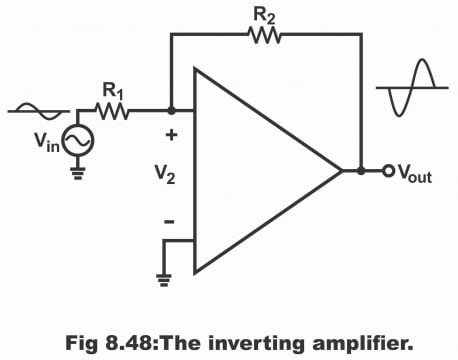How can this negative feedback strengthens cumulative voltage gain? It can be understood in this way. That if open loop voltage gain (AOL) rises due to some reason, output voltage will increase and inverting input will also have more feedback. These inverted feedback voltages received on input reduce V2. Thus, V2 will experience a decline despite an increase in AOL. And thus, very little increase occurs in final output compared to an increase in output without negative feedback. Thus, the total value of an increase in output voltage is so small, that it is often ignored.

When voltages on an inverting input are positive, Vout starts moving towards negative side. As these negative voltage feedbacks are applied on inverting input via resistor R2, therefore these voltages strive to abolish changes taking place in Vin. In fact, feedback signal cannot totally eliminate an input signal, because if such a phenomenon becomes possible, we will not be able to get any feedback signal. However, in spite of this, feedback signal minimizes the impact of an input signal to a great extent. Remember that voltages on an operational amplifiers’ inverting input are practically zero no matter what ever occurs in Vin. This task is performed by feedback voltage.

### Virtual Ground

Virtual ground is such a point on circuit which is located on ground potential (i.e. zero volts), however it is not connected to the ground. In figure 3.49, its three examples have been shown. In every condition, point A is on zero volts. However, it is isolated from the ground. The determination of voltage on point A is done via V1, V2 and resistor R1 and R2. If a volt meter is fitted between point A and ground, the volt meter will present a zero reading, though point A is isolated from the ground. Similarly, in case of amplifier Vi and Vout and resistors R1 and R2 keep the voltage of inverting input on zero volt as is clear in the figure 8.50. That’s the reason inverting input of an operational amplifier is considered as a virtual ground.

Figure 8.49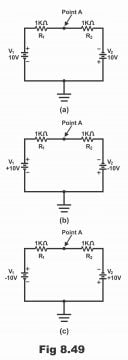Thus, node which is at zero potential with respect to the ground, however mechanically not grounded, is called a virtual ground. Two types of grounds are normally used in electronic circuits. First type is called mechanical ground. Under this method, a node is connected with ground practically via a wire. Mechanical ground shorts node with the ground on all frequencies. Second type of ground is known as AC ground, which is normally denoted by means of a bypass capacitor. As capacitor’s capacitive reactance is low on high frequencies, therefore this type of ground operates only on high frequencies. Remember that both types of grounds facilitate /transmit current from node to ground.

Virtual ground differs from the two types of ground as mentioned above. No path is provided to the ground from node in this type of ground, despite this node potential remains zero. Remember that a virtual ground is only grounded for voltage but not for current i.e. it operates as a half ground. It has been denoted by dotted lines in figure 8.50. The analysis of an inverting amplifier and its related circuit can quite easily be done with the help of a virtual ground.

Figure 8.50 – the concept of a virtual ground: shorted to voltage but open to current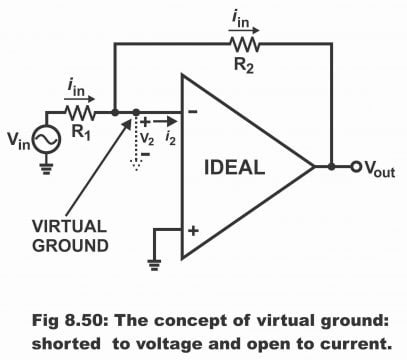The concept of a virtual ground depends on an ideal amplifier (i.e. in order to consider the concept of a virtual ground, operational amplifier is considered being an ideal one) When an operational amplifier is treated as an ideal amplifier, in such a situation its open loop voltage gain and its input resistance is considered as being infinite. Thus, the following typical characteristics can be derived from an inverting amplifier.

1). As input resistance (Rin) is infinite, therefore, i2 value is zero

2). As open loop gain (AOL) is infinite, therefore, V2 value is zero

As the value of i2 is zero (as is clear from the picture), therefore current passing through the feedback resistor (R2), is equal to input. Further, as the value of V2 is zero, therefore the virtual ground as shown in the figure implies that inverting input function as a ground for the voltage, however for current it operates as an open (i.e. it does not function as a ground for current). It has thus become evident that virtual ground tends to be of an exceptional type. It works like half of a ground due to being short for the voltage and open to current. That’s the reason it has been expressed as dashed lines between inverting input and ground in the figure. The meaning of these dashed lines is that no current flows towards the ground.

### Voltage Gain

In figure 8.51, a virtual ground has been shown on inverting input. In such a condition, right end of R1 is voltage ground. Thus, the value of input voltage will be as follows:

Vin = iin R1

Similarly, left end of R2 is voltage ground, thus the value of output voltage will be as below:

Vout = iin R2

Voltage gain results by dividing Vout by Vin, which equals the following simple equation.

Vout/Vin = iin R2/ iin R1 = ACL =R2/R1

Here, ACL means close loop gain. Due to negative feedback, close loop gain (ACL) is always less than open loop gain (AOL). Thus, close loop gain equals the mutual ratio between feedback resistance R2 and input resistance R1. For example, if R1 = 1KΩ and R2 = 50KΩ, the value of close loop gain will be 50. Due to heavy negative feedback, this close loop voltage gain tends to be quite stable. If AOL changes as a result of some change temperature, changes in supply voltage take place, by means of which ACL value still remains closer to 50.

Figure 8.51- inverting amplifiers has same current through both resistors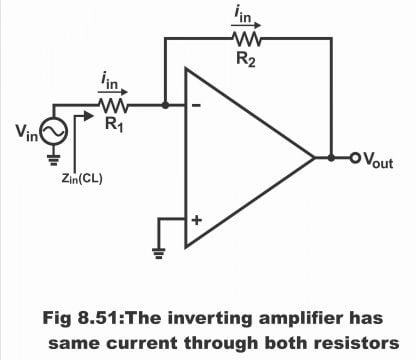#### Explanation of Voltage Gain

In other words, current flows through R1 by means of input signal Vi. As voltage of an operational amplifier on an inverting input tends to be zero, therefore Vi must fully drop in R1, as result input current iin becomes as follows:

Iin= Vi/ R1 … 1

Output voltage (Vout) is inverted with respect to phase or polarity of input voltage (Vin). Due to these voltages, feedback current, which equals iin, transmits through feedback resistor R2 (i.e. same or symmetrical current transmits through R1 and R2). The left side of resistor R2 is at zero volts whereas the right side is on Vout. Thus, the value of feedback current is as below:

iin = VOUT/R2 … 2

The negative sign reflects that there is a phase difference of 180˚ between Vout and Vin. Thus, no current can penetrate into the inverting input of an operational amplifier, nor any current flows out from it.

Comparing equation 1 and 2,

Vi/Ri = Vout/ R2

Arranging the above equation,

Vout/Vin = – R2/R1

Remember that the ratio Vout/ Vin reflects close loop gain

Or ACL = AV = – R2/R1 … (where Av = voltage gain)

The negative sign shows that signal has become inverted. The above equation reveals that circuit gain can only be determined through the mutual ratio of R2 and R1.

### Input and Output Impedance

In certain applications, a designer requires specific input impedance. One of the biggest advantages of inverting amplifiers is that it can be set on any specific or required input impedance quite easily. As right of R1 is a virtual ground, therefore the value of close loop input impedance will become equal to the following:

Z in(CL) = R1

This impedance is the left end impedance of R1, as can be seen from the figure. For example, if input impedance is 2KΩ and the required value of close gain voltage is 50, the designer can achieve this objective via keeping the value of R1 at 2KΩ and R2 value 100KΩ.

Remember that input impedance of an inverting amplifier never exceeds non – inverting amplifier or voltage follower. However, its output impedance, equals non- inverting amplifier (i.e. usually very low).

Zout (CL) = Zout/ AL

Here AL means loop gain.

Next Topic: Summing Amplifier

Previous Topic: Difference Amplifier with Equation Example

For electronics and programming-related projects visit my YouTube channel.